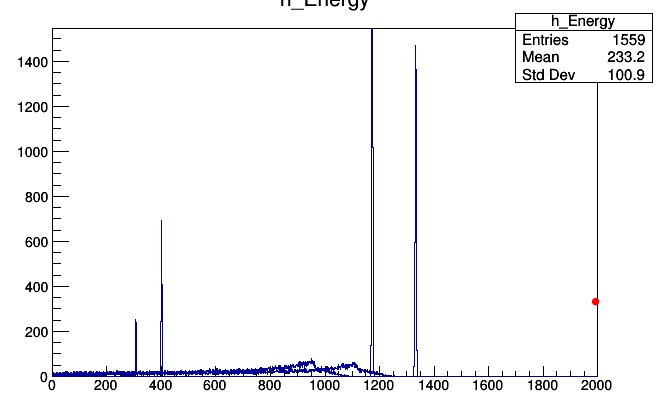# Draw Files on Same Canvas

ROOT Version: 6.19.02
Platform: Windows 10
Compiler: Visual Studio 2019

Hi there,

I have a root analysis file with multiple histograms that I would like to plot all on the same canvas. How would I go about this? I’m sure there must be a straightforward way of achieving this but I can’t figure it out. Any help would be much appreciated.

Looks like c1 are TCanvas, not histograms. What are the names of the histograms drawn when you double click on them? For 2 histos (TH1F, called h0 and h1), you could do something like:

``````void plotall() {
TFile *f=new TFile("myfile.root");
TCanvas *c0 = (TCanvas*)f->Get("c1;1");
TCanvas *c1 = (TCanvas*)f->Get("c1;2");
TH1F *h0 = (TH1F*)c0->GetListOfPrimitives()->FindObject("h0");
TH1F *h1 = (TH1F*)c1->GetListOfPrimitives()->FindObject("h1");
TCanvas *c2 = new TCanvas("c2");
c2->Divide(1,2);  // 1 column, 2 rows
c2->cd(1);
h0->Draw();
c2->cd(2);
h1->Draw();
//f->Close();
//delete f;
}
``````

For 4, you could do Divide(2,2) to have 2 cols, 2 rows, and then you will “cd” from 1 to 4.
If, on the other hand, you want all histos superposed, just don’t use Divide (nor cd()) and draw each one with the option “same”, except the first one:

``````  h0->Draw();
h1->Draw("same");
// ...
``````

but you will need to be careful with the bin ranges, as the canvas will keep the ranges of the first histogram you draw.

Yes sorry they are canvases. I only realised a little while after posting this. Thanks for the reply, I’ll look into applying this.

So I’m getting the following output, which is not exactly what I’m looking for.As you can see, this has superimposed each plot over each other, while what I want to do is effectively combine the data from each file, and then plot the full thing. Do you know if this is possible?